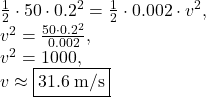## We have a toy gun with a spring constant of 50 N/m. The spring is compressed by 0.2 m. If you neglect friction and the mass of the spring, a

Question

We have a toy gun with a spring constant of 50 N/m. The spring is compressed by 0.2 m. If you neglect friction and the mass of the spring, at what speed will a 2 g projectile be ejected from the gun

in progress 0
3 months 2021-07-29T14:28:18+00:00 1 Answers 16 views 0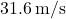Explanation:

The elastic potential energy of a spring is given by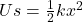, where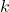is the spring constant of the spring and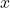is displacement from point of equilibrium.

When released, this potential energy will be converted into kinetic energy. Kinetic energy is given by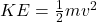, where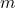is the mass of the object and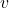is the object’s velocity.

Thus, we have: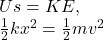Substituting given values, we get: